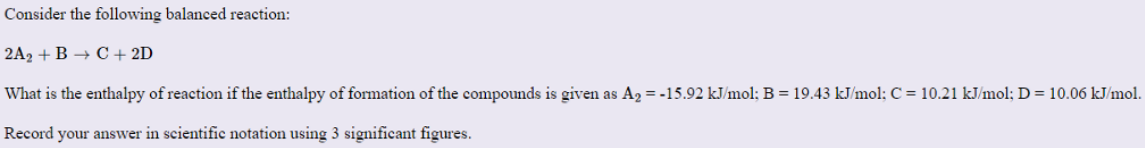# Problem: Consider the following balanced reaction: 2A 2 + B --&gt; C + 2D What is the enthalpy of reaction if the enthalpy of formation of the compounds is given as A 2 = -15.92 kJ/mol; B = 19.43 kJ/mol: C = 10.21 kJ/mol; D = 10.06 kJ/mol. Record your answer in scientific notation using 3 significant figures.

###### FREE Expert Solution
88% (256 ratings)###### Problem Details

Consider the following balanced reaction: 2A 2 + B --> C + 2D

What is the enthalpy of reaction if the enthalpy of formation of the compounds is given as A 2 = -15.92 kJ/mol; B = 19.43 kJ/mol: C = 10.21 kJ/mol; D = 10.06 kJ/mol. Record your answer in scientific notation using 3 significant figures.